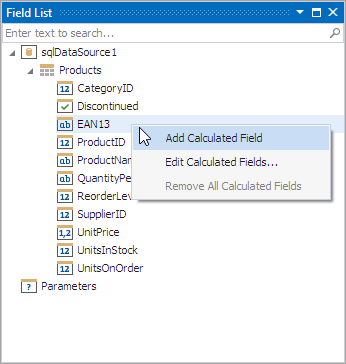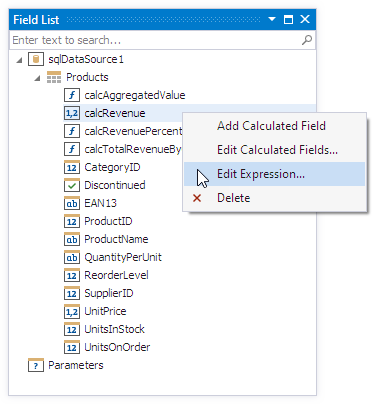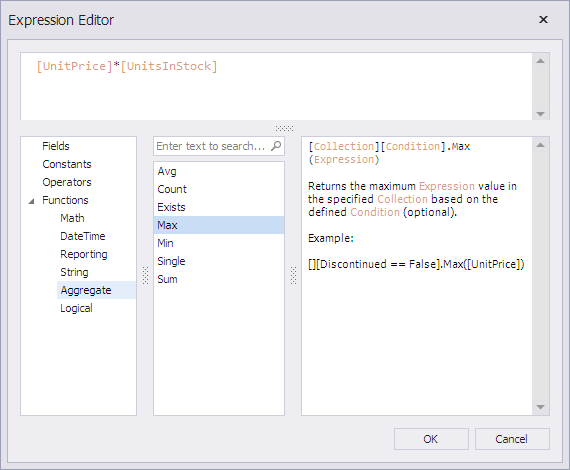20.2
20.1
19.2
19.1
18.2
18.1
17.2

# Calculated Fields Overview

Calculated fields are primarily used in data-aware reports when using both standard data binding and mail merge. Calculated fields allow you to pre-process a report's input data, based on a certain expression. So, using calculated fields allows you to apply complex expressions to one or more data fields that are obtained from your report's underlying data source. Moreover, you can both group and sort your report data based on a calculated field's value.

## Calculated Fields Overview

Calculated fields are represented by instances of the CalculatedField class residing in the CalculatedFieldCollection. Their collection is accessed via a report's XtraReport.CalculatedFields property.

At design time, they are easily managed via the Field List. For details, refer to Use Calculated Fields.The value of a calculated field is obtained by evaluating its expression, which is specified by its CalculatedField.Expression property's text. The Expression Editor can be used to visually construct a calculated field's expression. In the Field List, it is invoked by right-clicking a calculated field and choosing the Edit Expression... item in the invoked popup menu.Construct the required expression in the invoked Expression Editor. You can use data fields, report parameters, predefined constants as well as various date-time, logical, math and string functions.##### NOTE

The Expression Editor displays only those data fields that are obtained from a data source specified by the CalculatedField.DataSource and CalculatedField.DataMember property values.

Finally, you can group and sort your report data based on the calculated field values. For an example of this task, refer to Group Data by Days of the Week.

For tasks that for some reason cannot be solved using calculated fields, the XRControl.EvaluateBinding event was introduced, which allows you to perform custom calculations over data obtained from a control's data source.

## Expression Syntax

A data field is inserted into the expression's text using its name in [square brackets], and parameters are inserted using the "Parameters." prefix before their names.

A calculated field's expression can evaluate the values of other calculated fields if you make sure to avoid circular references.

##### NOTE

When creating calculated fields, avoid dots in their names, because XtraReports uses them to address data source members.

Date-time constants must be wrapped in hashtags (#) (e.g., [OrderDate] >= #1/1/2009#). To represent a null reference (one that does not refer to any object), use a question mark (e.g., [Region] != ?). To denote strings, use apostrophes ('), otherwise an error will occur.

To embed an apostrophe into an expression's text, it should be preceded by another apostrophe (e.g., 'It''s sample text').

The type of a value returned by a calculated field is defined by its CalculatedField.FieldType property. All available types are listed in the FieldType enumeration.

If a calculated field expression involves the use of different types, it is necessary to convert them to the same type (e.g., Max(ToDecimal([Quantity]),[UnitPrice]))

Although a value that is returned by a calculated field is usually converted to a string (to be displayed in a text-aware report control), it can return a value of any kind (if the CalculatedField.FieldType property is set to None). For example, if a database field contains an image, you can set a calculated field's expression to "=...", after which this calculated field can be bound to the XRPictureBox control.To construct a valid aggregate expression, use the following format, which consists of four parts.

[<Collection>][<Condition>].<Aggregate>(<Expression>)

• <Collection> - Specifies a collection against which an aggregated value should be calculated. It can be the relationship name in a case of a master-detail relationship, or the name of a collection property exposed by the target class. For example, [CategoriesProducts][[CategoryId]>5].Count(). Empty brackets [] indicate the root collection.
• <Condition> - Specifies a condition defining which records should participate in calculating an aggregate function. To obtain an aggregated value against all records, delete this logical clause along with square brackets (for example, [].Count()).
• <Aggregate> - Specifies one of the available aggregate functions listed in the Aggregate enumeration.
• <Expression> - Specifies an expression evaluating values to be used to perform calculation. For example, [][[CategoryID] > 5].Sum([UnitPrice]*[Quantity]). The Count function does not require field values to count the records, so leave the round brackets empty for this function.

You can refer to the currently processed group using the Parent Relationship Traversal Operator ('^'). This allows you to calculate aggregates within groups using expressions like the following: [][[^.CategoryID] == [CategoryID]].Sum([UnitPrice]).